GRID Statement
GRID grid-options </ option> ;

The GRID statement specifies the grid of spatial locations at which to perform the simulations. A single GRID statement is required and is applied to all SIMULATE statements. Specify the grid in one of the following three ways:

• Specify theand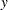coordinates explicitly for a grid in two dimensions.

• Specify the NPTS= option in addition to theandcoordinates to define a grid of individual points or in one dimension.

• Specify the coordinates by using a SAS data set for a grid of individual points or in one dimension.

The GRID statement has the following grid-options:

NPTS=number
NPTS=ALL

controls specification of a grid in one dimension or a grid of individual simulation locations.

When you specify the NPTS=number option and the coordinates of two points in the GRIDDATA= data set or in both the X= and Y= options, you request a linear simulation grid. Its direction is across the line defined by the specified points. The grid size is equal to the number of points that you specify in the NPTS= option, where number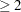.

When you specify the NPTS=ALL option and the coordinates for any number of points in the GRIDDATA= data set or in each of the X= and Y= options, the SIM2D procedure performs simulation only at the specified individual locations. Use the NPTS=ALL option to examine a set of individual points anywhere on the XY plane or to specify a custom grid in one dimension.

If the number ofcoordinates and the number ofcoordinates in the X= and Y= options, respectively, are different, then the NPTS= option is ignored; in that case, a two-dimensional grid is used according to the specified X= and Y= options.

If you specify a simulation grid with any number of points other than two in the GRIDDATA= data set, then the option NPTS=ALL has the same effect as omitting the NPTS= option.

X=number
X=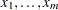X=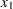to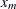X=toby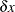specifies thecoordinate of the grid locations.

Y=number
Y=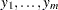Y=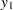to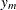Y=toby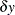specifies thecoordinate of the grid locations.

Use the X= and Y= options of the GRID statement to specify a grid in one or two dimensions, or a grid of individual simulation locations.

For example, the following two GRID statements are equivalent:

```GRID X=1,2,3,4,5  Y=0,2,4,6,8,10;
```
```GRID X=1 TO 5 Y=0 to 10 by 2;
```

In the following example, the first GRID statement produces a grid in two dimensions. The second statement produces simulation output only for the four individual points at the locations (1,0), (2,5), (3,7), and (4,10) on the XY plane.

```GRID X=1 TO 4 Y=0,5,7,10;
```
```GRID X=1 TO 4 Y=0,5,7,10 NPTS=ALL;
```

In the next example, the first GRID statement specifies a 2-by-2 grid in two dimensions. The second GRID statement specifies a linear grid of eight points. The grid is in the direction of the line defined by the specified points (2,8) and (3,5) on the XY plane and it extends between these two points.

```GRID X=2,3 Y=8,5;
```
```GRID x=2,3 Y=8,5 NPTS=8;
```

The last example shows a GRID statement that specifies a linear grid made of seven points across the Y axis. In this case, the syntax is sufficient to fully define a linear grid without the NPTS= option.

```GRID X=5 Y=3 TO 9;
```

To specify grid locations from a SAS data set, you must provide the name of the data set and the variables that contain the values of theandcoordinates.

GRIDDATA=SAS-data-set
GDATA=SAS-data-set

specifies a SAS data set that contains theandgrid coordinates. Use the GRIDDATA= option of the GRID statement to specify a grid in one dimension or a grid of individual simulation locations.

XCOORD=(variable-name)
XC=(variable-name)

gives the name of the variable that contains thecoordinate of the grid locations in the GRIDDATA= data set.

YCOORD=(variable-name)
YC=(variable-name)

gives the name of the variable that contains thecoordinate of the grid locations in the GRIDDATA= data set.

You can specify the following option in the GRID statement after a slash (/):

LABEL <(suboption)> = (character-list)

specifies labels to tag grid points in simulation plots when you use grids in one dimension. You can specify one or more such labels as quoted strings in the character-list.

When the number of labels in the character-list exceeds the number of points in your grid, the labels in the list are used sequentially and any labels in excess are ignored. When the number of labels in the character-list is smaller than the number of points in your grid, the behavior is as follows:

• If an area map is included in the simulation plot, then blank labels are assigned to the remaining nonlabeled grid points on the map.

• For the simulation band and box plots, the coordinates of nonlabeled grid points are automatically assigned as their labels.

If the grid points are colinear and the horizontal axis displays distance, then two labels appear by default in the simulation plot. These are assigned to the first and the last points of the grid to help identify the ends of the linear grid segment on the plot map. This label pair is shown only when the plot includes an area map. Specifically, the two labels appear when you request simulation band plots, or simulation box plots for which you specify the SIM(SHOWD) suboption, if applicable. The two labels do not appear if you specify explicitly the NOMAP suboption in the PLOTS=SIM option.

The two labels have default values, unless you choose to specify your own labels with the LABEL= option. If you specify more than two labels in the character-list under these conditions, then only the first and last labels in the list are used; any additional labels in between are ignored.

The LABEL= option has the following suboption:

ALL

specifies that all individual points in the grid are assigned sequentially the labels you specify in the LABEL(ALL)= option when the SIM(SHOWD) suboption is applicable and specified in a simulation box plot. In all other cases, the ALL suboption is ignored.

The ALL suboption enables you to override the default behavior when the SIM(SHOWD) suboption is specified (the default behavior is to display labels only for the first and last grid points). As a result, you can use the ALL suboption to label grid grid points in both conditional and unconditional simulation tasks regardless of whether you specify the NOMAP suboption in the PLOTS=SIM option.

The LABEL= option is ignored when you produce simulation plots of grids in two dimensions.